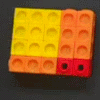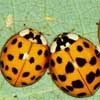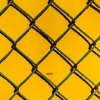# Resources tagged with: Expanding and factorising quadratics

Filter by: Content type:
Age range:
Challenge level:

### There are 26 results

Broad Topics > Algebraic expressions, equations and formulae > Expanding and factorising quadratics##### Age 14 to 16 Challenge Level:

Can you find out what is special about the dimensions of rectangles you can make with squares, sticks and units?### Polar Flower

##### Age 16 to 18 Challenge Level:

This polar equation is a quadratic. Plot the graph given by each factor to draw the flower.### Parabella

##### Age 16 to 18 Challenge Level:

This is a beautiful result involving a parabola and parallels.### Powerful Factors

##### Age 16 to 18 Challenge Level:

Use the fact that: x²-y² = (x-y)(x+y) and x³+y³ = (x+y) (x²-xy+y²) to find the highest power of 2 and the highest power of 3 which divide 5^{36}-1.### Always Perfect

##### Age 14 to 16 Challenge Level:

Show that if you add 1 to the product of four consecutive numbers the answer is ALWAYS a perfect square.### Geometric Parabola

##### Age 14 to 16 Challenge Level:

Explore what happens when you draw graphs of quadratic equations with coefficients based on a geometric sequence.### Fair Shares?

##### Age 14 to 16 Challenge Level:

A mother wants to share a sum of money by giving each of her children in turn a lump sum plus a fraction of the remainder. How can she do this in order to share the money out equally?### Perfectly Square

##### Age 14 to 16 Challenge Level:

The sums of the squares of three related numbers is also a perfect square - can you explain why?### Number Rules - OK

##### Age 14 to 16 Challenge Level:

Can you convince me of each of the following: If a square number is multiplied by a square number the product is ALWAYS a square number...### Telescoping Functions

##### Age 16 to 18

Take a complicated fraction with the product of five quartics top and bottom and reduce this to a whole number. This is a numerical example involving some clever algebra.### 2-digit Square

##### Age 14 to 16 Challenge Level:

A 2-Digit number is squared. When this 2-digit number is reversed and squared, the difference between the squares is also a square. What is the 2-digit number?### Never Prime

##### Age 14 to 16 Challenge Level:

If a two digit number has its digits reversed and the smaller of the two numbers is subtracted from the larger, prove the difference can never be prime.### Fibonacci Factors

##### Age 16 to 18 Challenge Level:

For which values of n is the Fibonacci number fn even? Which Fibonnaci numbers are divisible by 3?### Poly Fibs

##### Age 16 to 18 Challenge Level:

A sequence of polynomials starts 0, 1 and each poly is given by combining the two polys in the sequence just before it. Investigate and prove results about the roots of the polys.### Novemberish

##### Age 14 to 16 Challenge Level:

a) A four digit number (in base 10) aabb is a perfect square. Discuss ways of systematically finding this number. (b) Prove that 11^{10}-1 is divisible by 100.### Common Divisor

##### Age 14 to 16 Challenge Level:

Find the largest integer which divides every member of the following sequence: 1^5-1, 2^5-2, 3^5-3, ... n^5-n.### Code to Zero

##### Age 16 to 18 Challenge Level:

Find all 3 digit numbers such that by adding the first digit, the square of the second and the cube of the third you get the original number, for example 1 + 3^2 + 5^3 = 135.### Two Cubes

##### Age 14 to 16 Challenge Level:

Two cubes, each with integral side lengths, have a combined volume equal to the total of the lengths of their edges. How big are the cubes? [If you find a result by 'trial and error' you'll need to. . . .### Multiplication Magic

##### Age 14 to 16 Challenge Level:

Given any 3 digit number you can use the given digits and name another number which is divisible by 37 (e.g. given 628 you say 628371 is divisible by 37 because you know that 6+3 = 2+7 = 8+1 = 9). . . .### Composite Notions

##### Age 14 to 16 Challenge Level:

A composite number is one that is neither prime nor 1. Show that 10201 is composite in any base.### Plus Minus

##### Age 14 to 16 Challenge Level:

Can you explain the surprising results Jo found when she calculated the difference between square numbers?### Always Two

##### Age 14 to 18 Challenge Level:

Find all the triples of numbers a, b, c such that each one of them plus the product of the other two is always 2.### Unit Interval

##### Age 16 to 18 Short Challenge Level:

Can you prove our inequality holds for all values of x and y between 0 and 1?### Spot the Difference

##### Age 16 to 18 Short Challenge Level:

If you plot these graphs they may look the same, but are they?### Spinners

##### Age 16 to 18 Challenge Level:

How do scores on dice and factors of polynomials relate to each other?### Finding Factors

##### Age 14 to 16 Challenge Level:

Can you find the hidden factors which multiply together to produce each quadratic expression?